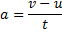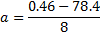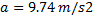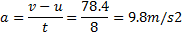# Velocity & acceleration

• Barclay

#### Barclay

Missing homework template due to originally being posted in other forum.
This is not a homework question so I have posted here.
<<Mentor note: This question is homework-like and therefore belongs in the homework forums. Please also refrain from excessive use of colours and font sizes.>>

The question relates to velocity and acceleration. I think the answer of the book is incorrect.

Description: A coin is rolled along the balcony edge at the steady speed of 0.46 m/s before falling off the edge after 2.4 seconds. It then accelerates due to gravity and hits the ground after 8 seconds at a speed of 78.4 m/s. Assume that no air resistance acts on the coin.

The first part of the question asks how far the coin rolls before falling off the edge of the balcony. I got this part correct with the answer 1.1 m.

The second part asks: calculate the acceleration of the coin during its fall.

My calculation is:The book just writesSo the book seems to have forgotten that the initial velocity of the coin was 0.46 m/s. Have they just assumed that the coin started from 0 m/s velocity or am I missing something? Please advise. Thank you

Last edited by a moderator:
Seems to me the velocity was .46 horizontally then vertically it starts at 0.

or am I missing something?
Yes, you are. Remember that velocity is a vector.

velocity is a vector.

So that means the vertical velocity is 0m/s at onset (as jerromyjon said) and it is only the horizontal velocity that is 0.46 m/s ??

That's right.

Thanks all. This forum is great.

Don't let the "Thanks all" stop you ... keep posting if there's more to say

Well, if you care for a follow-up question to test your new understanding, perhaps you could try answering how far from the edge of the balcony the coin lands?

Well, if you care for a follow-up question to test your new understanding, perhaps you could try answering how far from the edge of the balcony the coin lands?

I think this is going to involve triangles, sine and cosine etc. That's beyond me at the moment. Maybe in a few months be able I'll look at this follow up question again

I think this is going to involve triangles, sine and cosine etc. That's beyond me at the moment. Maybe in a few months be able I'll look at this follow up question again

No, you should be able to do it pretty much in your head. What is the horizontal velocity of the coin when it leaves the balcony? Does this change as it falls? How long does it fall?